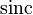# Sinc function

(diff) ← Older revision | Latest revision (diff) | Newer revision → (diff)
This article is about a particular function from a subset of the real numbers to the real numbers. Information about the function, including its domain, range, and key data relating to graphing, differentiation, and integration, is presented in the article.
View a complete list of particular functions on this wiki
For functions involving angles (trigonometric functions, inverse trigonometric functions, etc.) we follow the convention that all angles are measured in radians. Thus, for instance, the angle of$90\,^\circ$ is measured as$\pi/2$.

## Definition

This function, denoted$\operatorname{sinc}$, is defined as follows:$\operatorname{sinc} x := \left\lbrace \begin{array}{rl} 1, & x = 0 \\ \frac{\sin x}{x} & x \ne 0\end{array}\right.$

## Graph

Below is a graph of the function for the domain restricted to$[-3\pi,3\pi]$:

The picture is a little unclear, so we consider an alternative depiction of the graph where the$x$-axis and$y$-axis are scaled differently to make it clearer: